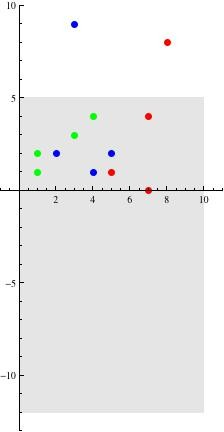Are any of these correct? Give example of a function using a set of at least 4 ordered pairs. The domain will be any four integers between 0 and +10. The range will be any four integers between -12 and 5.

None of the offered choices is correct.
(a) the range includes 9, which is not between -12 and 5.
(b) there are two different values given for (1, ), so the relation does not meet the definition of a function
(c) the range includes 8, which is not between -12 and 5. Also, there are two different values given for (7, ), so the relation does not meet the definition of a function.

Refer to the graph below. You want 4 points inside the gray area such that no two are above/below each other. The blue, green, red points are the sets you have provided here.thanked the writer.
Oddman commented
I apologize for changing my answer "after the fact." I thought a graphic might be helpful. In creating it, I saw that "red" wasn't a function, either.
linda butch commented
That's ok I appreciate the help and the graft helped but I'm still confused. Are the examples you gave me wrong? I have tried every way I know how to get this but I'm still lost.
Oddman commented
The full example I gave is correct. (1, -3), (2, -2), (3, -1), (4, 0) is a suitable answer. Print the answer above. Throw 4 darts at the gray rectangle. List the points where they hit (using integer values). That's your answer. (Make sure no two have the same first number.) It is too simple, perhaps.